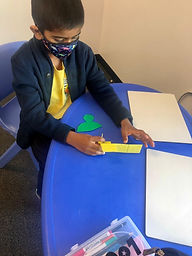## Mr. Rodney

### Target 1​

###### Lesson Type:

New

Geometry

:

Shape

Classify 2D and 3D figures based on the basic properties of shapes.

###### 1:

Classify shapes based on side measurements (2D figures).

###### 2:

Identify the properties of geometric nets and relate them to solid figures.

3rd

###### Vocabulary:

Polygons, 2- and 3-Dimensions, Net, Prism, Cube, Cylinder, Pyramid

Activities:

• Reviewed and discussed vocabulary terms, defining them using words that are accurate yet meaningful to them.
• Explored 3D figures and their 2D nets, understanding how the relate to each other.
• Found real world objects that are examples of each type of 3D figure.
• Matched net to its corresponding figure.### Home Exploration

###### Challenge Problem:

Alvin, Simon, and Theodore each have a box of shapes they can put together and make a 3D figure. Read the clues to figure out which 3D figure each boy made

###### Challenge Data:

Alvin: 4 triangles
Simon: 2 congruent circles and 1 rectangle
Theodore: 2 congruent squares and 4 rectangles

###### Guiding Questions:
1. · Review shapes and vocabulary, i.e., prism, pyramid, cube, cylinder, and net
2. · What is the difference between a pyramid and a prism? A pyramid comes to a point when sitting on its base and a prism is flat. Also, a pyramid uses more triangles while a prism uses more rectangles.
3. · Have shape pieces or paper and pencil available to help your child visualize and/or create the nets or 3D figures.
4. · Allow your child to be creative and see what composite 3D shapes they can create.

Alvin: triangular pyramid
Simon: cylinder
Theodore: rectangular prism## Absent Students:

### Target 2

:

###### 1:

Be introduced to a variety of computation strategies for subtracting within 1,000 and select a strategy that works best.

###### 2:

Understand why the standard algorithm for subtraction is based on place-value.

###### 3:

Understand that subtraction equations can be written both vertically and horizontally.

###### 4:

Understand how to regroup based on place-value understandings (i.e., ten ones can be composed into one ten – ten tens can be composed into one hundred).

###### 5:

Understand that the same process is utilized to subtract three or more whole numbers that is used to subtract only two numbers.

3rd

###### Vocabulary:

Subtract, Minus, Minuend, Subtrahend, Difference

Activities:

• Reviewed how to subtract using the standard algorithm with 2- and 3-digit numbers.
• Applied the process to subtraction problems with multiple subtrahends. For example, 86-12-45-22=__.
• Compared these types of problems to addition and discovered that with addition, one can simply add all the digits within the place values while with subtraction, each number needs to be subtracted individually.
• Given a "color by number" style worksheet, students solved for the difference for each section and colored the part based on the answer's corresponding color.### Home Exploration

###### Guiding Questions:### Target 3

:

###### Vocabulary:

Activities:### Home Exploration